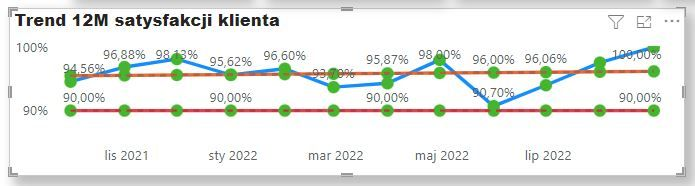cancel
Showing results for
Did you mean:Helper I

## Calculated TREND line but wrong displayed X axis

Hello Team,

I trying to show trend line on my graphs using tweak linear regresion DAX code as below:

``````Score Trend =
VAR Known =
FILTER (
SELECTCOLUMNS (
ALLSELECTED ( 'COQ Calendar'[WC_Month] ),
"Known[X]", 'COQ Calendar'[WC_Month],
"Known[Y]", [Score]
),
AND (
NOT ( ISBLANK ( Known[X] ) ),
NOT ( ISBLANK ( Known[Y] ) )
)
)
VAR Count_Items =
COUNTROWS ( Known )
VAR Sum_X =
SUMX ( Known, Known[X] )
VAR Sum_X2 =
SUMX ( Known, Known[X] ^ 2 )
VAR Sum_Y =
SUMX ( Known, Known[Y] )
VAR Sum_XY =
SUMX ( Known, Known[X] * Known[Y] )
VAR Average_X =
AVERAGEX ( Known, Known[X] )
VAR Average_Y =
AVERAGEX ( Known, Known[Y] )
VAR Slope =
DIVIDE (
Count_Items * Sum_XY - Sum_X * Sum_Y,
Count_Items * Sum_X2 - Sum_X ^ 2
)
VAR Intercept =
Average_Y - Slope * Average_X

VAR MaxDate =
MAX ( 'DATE_DUP'[WC_Month] )
VAR MinDate =
DATE ( YEAR ( MaxDate ), MONTH ( MaxDate ) - 12, DAY ( MaxDate ) )

RETURN
CALCULATE (
SUMX (
DISTINCT ( 'COQ Calendar'[WC_Month] ),
Intercept + Slope * 'COQ Calendar'[WC_Month]
),
FILTER ( 'COQ Calendar', 'COQ Calendar'[WC_Month] > MinDate && 'COQ Calendar'[WC_Month] <= MaxDate )
)``````

When drag & drop this measure to my graph then result of above measure if covering real results as below:This happened becasue of applied hierarchy of dates:When I changing this to remove to WC_Month:Trend line seems to correct but X axis is moved about 1 month to right which means data from August 2022 are displayed as September 2022:Data are filterd based DATE_DUP table which is created with below code:

``DATE_DUP = DISTINCT('COQ Calendar'[WC_Month])``

But table "COQ Calendar'[WC_Month] from below code:

``WC_Month = EOMONTH('COQ Calendar'[Year] & " " & 'COQ Calendar'[Month_2],0)``

1 ACCEPTED SOLUTIONCommunity Support

Do you use Month End Date column on X axis? If so, it will move one month right to the next month because Power BI will adjust the axis display values automatically according to the width of the visual. As the month end date is near the start date of next month, it moves to the next month.

To avoid this, it is recommended to use a date column like Month Start date on the axis. Based on your current DAX, you can try

``WC_Month = EOMONTH('COQ Calendar'[Year] & " " & 'COQ Calendar'[Month_2],-1) + 1``

Best Regards,
Community Support Team _ Jing
If this post helps, please Accept it as Solution to help other members find it.

2 REPLIES 2Helper I

I did the same ba changing EOMONTH function to EDATE function.

``WC_Month = EDATE('COQ Calendar'[Year] & " " & 'COQ Calendar'[Month_2],0)``Community Support

Do you use Month End Date column on X axis? If so, it will move one month right to the next month because Power BI will adjust the axis display values automatically according to the width of the visual. As the month end date is near the start date of next month, it moves to the next month.

To avoid this, it is recommended to use a date column like Month Start date on the axis. Based on your current DAX, you can try

``WC_Month = EOMONTH('COQ Calendar'[Year] & " " & 'COQ Calendar'[Month_2],-1) + 1``

Best Regards,
Community Support Team _ Jing
If this post helps, please Accept it as Solution to help other members find it.